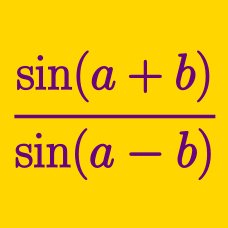Geometry

# Sum to Product Trigonometric Identities

Which of the following is equal to $\cos114^\circ+\cos44^\circ?$

Which of the following is equal to $\cos88^\circ-\cos42^\circ?$

Which of the following is equal to $\sin60^\circ+\sin16^\circ?$

Evaluate $16\sin120^\circ+8\cos105^\circ+16\sin60^\circ+8\cos15^\circ.$

Which of the following expressions is equal to $\sin{36 ^\circ} + \sin{24 ^\circ} ?$

×